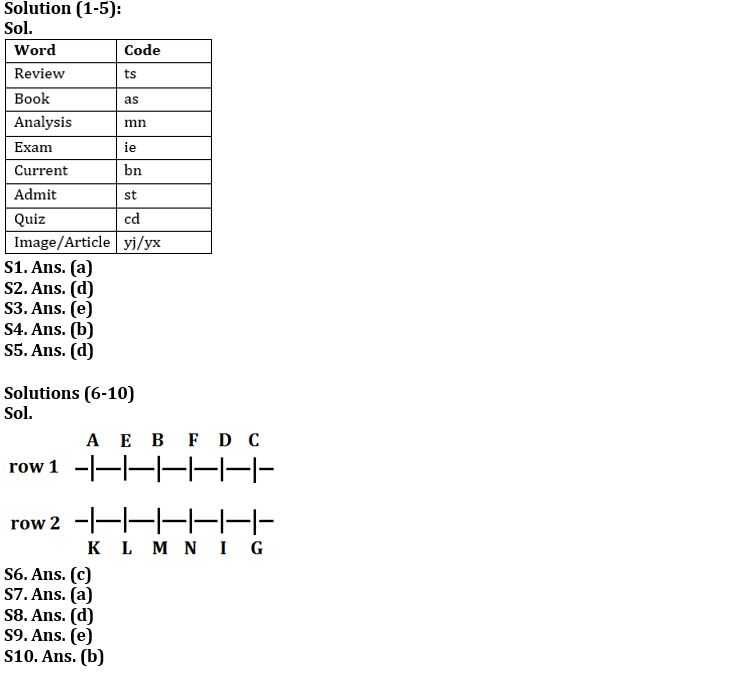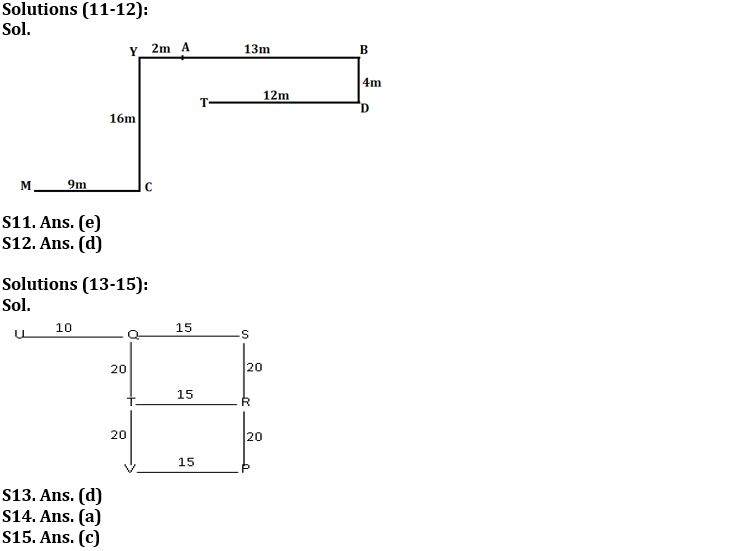Latest Banking jobs   »

# Reasoning Ability Quiz For SBI Clerk Prelims 2022- 10th September

Directions (1-5): Study the following information carefully and answer the given questions.
In a certain code language,
‘Review exam analysis book’ is coded as ‘ts ie mn as’
‘Current admit exam analysis’ is coded as ‘mn bn st ie’
‘Current quiz analysis book’ is coded as ‘cd as mn bn’
‘Review book image article’ is coded as ‘ts as yj yx’

Q1. Which of the following is the code for ‘admit’?
(a) st
(b) bn
(c) ie
(d) mn
(e) None of these

Q2. Which of the following quiz is coded as ‘bn’?
(a) quiz
(b) book
(d) Current
(e) None of these

Q3. What may be the code of ‘quiz orange’?
(a) st ie
(b) cd mn
(c) ie bn
(d) mn ie
(e) cd qw

Q4. Which of the following code is coded for ‘Exam’?
(a) mn
(b) ie
(c) bn
(d) cd
(e) None of these

Q5. What will be the code of ‘article?
(a) yj
(b) as
(c) yx
(d) Either (a) or (c)
(e) None of these

Directions (6-10): Read the given information carefully and answer the questions:
Twelve Persons are sitting in two parallel rows -A, B, C, D, E and F are sitting in row 1 facing south and I, G, K, L, M and N are sitting in row 2 facing north. Therefore, in the given seating arrangement each member seated in a row faces another member of the other row. L sits third to the left of I. F does not face I. Neither L nor I sits at extreme ends. G sits at one of the extreme ends. Only two people sit between G and M. F is not an immediate neighbor of E. M does not face F. Two persons sit between B and C. F does not sit at any of the extreme ends. E faces L. C is not an immediate neighbor of E. A does not face I. K sits left of N.

Q6. Who among the following sits diagonally opposite to C?
(a) G
(b) F
(c) K
(d) I
(e) None of these

Q7. Who among the following sits third to the left of E?
(a) D
(b) F
(c) A
(d) C
(e) None of these

Q8. Who among the following faces M?
(a) A
(b) F
(c) C
(d) B
(e) E

Q9. Four of the following five form a group, which among the following does not belong to this group?
(a) G, F
(b) N, E
(c) M, A
(d) I, B
(e) L, A

Q10. How many persons sit between N and K?
(a) Three
(b) Two
(c) None
(d) One
(e) More than Three

Direction (11-12): Study the given information and answer the following question.
Point A is 13m west of point B. Point D is 4m south of point B. Point C is east of 9m of point M. Point T is 12m west of point D. Point Y is 16m north of point C. Point Y is 2m west of point A.

Q11. What is the direction of C with respect of T?
(a) North-west
(b) South
(c) North-east
(d) West
(e) None of these

Q12. In which direction of point A with respect to Point T?
(a) North
(b) North-east
(c) East
(d) North-west
(e) None of these

Directions (13-15): Study the following information carefully and answer the given questions.
Point S is 40 m towards the North of Point P. Point Q is 15 m towards the West of point S. Point U is 25 m towards the West of point S. Point T is 20 m towards the South of point Q. Point R is 15 m towards the East of point T. Point V is 15 m towards the West of point P.

Q13. Which of the following points are in a straight line?
(a) S, T, P
(b) T, V, R
(c) S, Q, V
(d) T, V, Q
(e) U, Q, R

Q14. P is in which direction with respect to R?
(a) South
(b) North
(c) East
(d) West
(e) Cannot be determined

Q15. If a person walks 10 m towards East from point U and then takes a right turn, which of the following points would he reach first?
(a) V
(b) S
(c) T
(d) P
(e) R

Solutions#### Congratulations!Download Hindu Review of October 2021: Free PDF